# RS Aggarwal Class 9 Solutions Chapter 9 - Quadrilaterals And Parallelograms

## RS Aggarwal Class 9 Chapter 9 - Quadrilaterals And Parallelograms Solutions Free PDF

A quadrilateral is a polygon which contains four side whereas, a parallelogram is a type of quadrilateral, whose pairs of opposite sides are parallel. One of the most common properties of all quadrilaterals is that sum of all the angles is 360 degrees. Some of the different types of quadrilaterals are:

1. Rhombus
2. Trapezium
3. Square
4. Parallelogram
5. Rectangle

Parallelograms have some unique properties such as:

• Opposite sides are congruent
• Opposite angles are congruent
• It has adjacent angles which are supplementary
• It has diagonals which bisect each other.

## Download PDF of RS Aggarwal Class 9 Solutions Chapter 9 – Quadrilaterals And Parallelograms

Students must practice these solutions to gain more marks in the exam. We have provided here all the exercise questions of RS Aggarwal Maths solution to help you to revise the complete syllabus. It is an important tool to prepare effectively for the Class 9 Maths examination. All the solutions are explained in a detailed step-by-step manner for the better understanding of the students.

Q.1: Three angles of a quadrilateral measure 56°, 115°, 84°. Find the measure of the fourth angle.

Sol:

Let, the measure of the fourth angle be X$X^{\circ}$

Since the sum of the angle of a quadrilateral is 360$360^{\circ}$, we have:

56 + 115 + 84 + X = 360

$\Rightarrow$ 255 + X = 360

$\Rightarrow$ X = 105

Hence, the measure of the fourth angle is 105$105^{\circ}$

Q.2: The angles of a quadrilateral are in the ratio 2 : 4 : 5 : 7. Find the angles.

Sol:

Let, A$\angle A$ = 2X$2X^{\circ}$

Then B=(4X)$\angle B = (4X)^{\circ}$

C=(5X)$\angle C = (5X)^{\circ}$ and

D=(7X)$\angle D = (7X)^{\circ}$

Since the sum of the angles of a quadrilateral is 360$360^{\circ}$, we have:

2X + 4X + 5X + 7X = 360$360^{\circ}$

$\Rightarrow$ 18X = 360$360^{\circ}$

$\Rightarrow$ X = 20$20^{\circ}$

Therefore, Angle A = 40$40^{\circ}$

Angle B = 80$80^{\circ}$

Angle C = 100$100^{\circ}$

Angle D = 140$140^{\circ}$

Q.3: In the adjoining figure, ABCD is a trapezium in which AB $\parallel$ DC. If ∠ A = 55°and ∠ B = 70°, find ∠ C and ∠ D.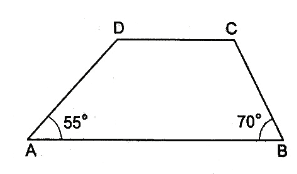Sol:

We have ABDC$AB\parallel DC$

Angle A and Angle D are the interior angles on the same side of the transversal line AD, whereas angle B and Angle C are the same interior angles on the same side of transversal line BC.

Now, Angle A + Angle D = 180$180^{\circ}$

$\Rightarrow$ D$\angle D$ = 180$180^{\circ}$A$\angle A$

Therefore, D$\angle D$ = 180$180^{\circ}$55$55^{\circ}$ = 125$125^{\circ}$

Again, B$\angle B$ + C$\angle C$ = 180$180^{\circ}$

$\Rightarrow$ C$\angle C$ = 180$180^{\circ}$B$\angle B$

Therefore, C$\angle C$ = 180$180^{\circ}$70$70^{\circ}$ = 110$110^{\circ}$

Q.4: In the adjoining figure, ABCD is a square and  ΔEDC$\Delta EDC$ is an equilateral triangle. Prove that (i) AB = BE, (ii) ∠ DAE = 15°.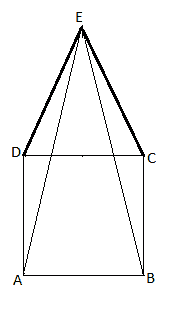Sol:

ABCD is a square in which AB = BC = CD = DA. ΔEDC$\Delta EDC$ is an equilateral triangle in which ED = EC = DC

EDC$\angle EDC$ = DEC$\angle DEC$ = DCE$\angle DCE$ = 60$60^{\circ}$.

To prove: AE = BE and DAE$\angle DAE$ = 15$15^{\circ}$

Proof: In ΔADE$\Delta ADE$ and ΔBCE$\Delta BCE$, we have:

AD = BC [Sides of a square]

DE = EC [Sides of an equilateral triangle]

ADE$\angle ADE$ = BCE$\angle BCE$ = 90$90^{\circ}$ + 60$60^{\circ}$ = 150$150^{\circ}$

ΔADE$\Delta ADE$ $\cong$ ΔBCE$\Delta BCE$

i.e., AE = BE

Now, ADE$\angle ADE$ = 150$150^{\circ}$

DA = DC [Sides of a square]

DC = DE [Sides of an equilateral triangle]

So, DA = DE

ΔADE$\Delta ADE$ and ΔBCE$\Delta BCE$ are isosceles triangles.

i.e., DAE$\angle DAE$ = DEA$\angle DEA$

= 12(180150)$\frac{1}{2}(180^{\circ}-150^{\circ})$

= 302$\frac{30^{\circ}}{2}$

= 15$15^{\circ}$

Q.5: In the adjoining figure, BM ⊥ AC and DN ⊥ AC. If BM = DN, prove that AC bisects BD.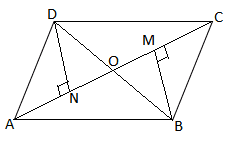Sol:

A quadrilateral ABCD, in which BM $\perp$ AC and DN $\perp$ AC and BM = DN

To prove: AC bisects BD, or DO = BO

Proof: Let, AC and BD intersect at O

Now, in ΔOND$\Delta OND$ and ΔOMB$\Delta OMB$, we have:

OND$\angle OND$ = OMB$\angle OMB$ (90$90^{\circ}$ each)

DON$\angle DON$ = BOM$\angle BOM$ (Vertically opposite angles)

Also, DN = BM (Given)

i.e., ΔOND$\Delta OND$ $\cong$ ΔOMB$\Delta OMB$ (AAS congruence rule)

Therefore, OD = OB (CPCT)

Hence, AC bisects BD

Q.6: In the given figure, ABCD is a quadrilateral in which AB = AD and BC = DC. Prove that:

(i) AC bisects ∠ A and ∠ C,  (ii) BE = DE, (iii)  ∠ ABC = ∠ADC.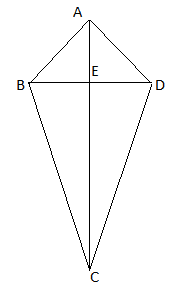Sol:

Given: ABCD is a quadrilateral in which AB = AD and BC = DC

(i) In ΔABC$\Delta ABC$ and ΔADC$\Delta ADC$, we have:

AB = AD (Given)

BC = DC (Given)

AC is common

i.e., ΔABC$\Delta ABC$ $\cong$ ΔADC$\Delta ADC$ (SSS Congruence rule)

Therefore, BAC$\angle BAC$ = DAC$\angle DAC$ and

BCA$\angle BCA$ = DCA$\angle DCA$ (By CPCT)

Thus, AC bisects A$\angle A$ and C$\angle C$

(ii) Now, in ΔABE$\Delta ABE$ and ΔADE$\Delta ADE$, we have:

AB = AD (Given)

BAE$\angle BAE$ = DAE$\angle DAE$ (Proven above)

AE is common.

Therefore, ΔABE$\Delta ABE$ $\cong$ ΔADE$\Delta ADE$ (SAS congruence rule)

$\Rightarrow$ BE = DE (By CPCT)

(iii) ΔABC$\Delta ABC$ $\cong$ ΔADC$\Delta ADC$ (Proven above)

Therefore, Angle ABC = Angle ADC (By CPCT)

Q.7: In the given figure, ABCD is a square and ∠PQR = 90°. If PB = QC = DR, prove that (i) QB = RC, (ii) PQ = QR (iii) ∠ QPR = 45°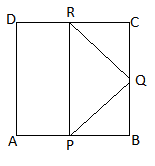Sol:

Let, ABCD be a parallelogram

∠ A = ∠ C and ∠ B = ∠ D (Opposite angles)

Let ∠ A be the smallest angle whose measure is x°

∴ ∠B = (2x – 30)°

Now, ∠ A + ∠ B = 180° (Adjacent angles are supplementary)

$\Rightarrow$ x + 2x – 30° = 180°

$\Rightarrow$ 3x = 210°

$\Rightarrow$ x = 70°

∴ ∠B = 2 x 70°- 30° = 110°

Hence, ∠ A = ∠ C = 70°; ∠ B = ∠ D = 110°

Q.8: If O is a point within a quadrilateral ABCD, show that OA + OB + OC + OD > AC + BD.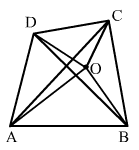Sol:

Let, ABCD be a quadrilateral whose diagonals are AC and BD and O is any point within the quadrilateral

Join O with A, B, C, and D

We know that the sum of any sides of a triangle is greater than the third side

So, in ΔAOC$\Delta AOC$, OA + OC > AC

Also, ΔBOD$\Delta BOD$, OB + OD > BD

Adding these inequalities, we get:

(OA + OC) + (OB + OD) > (AC + BD)

$\Rightarrow$ OA + OB + OC + OD > AC + BD

Q.9: In the adjoining figure, ABCD is a quadrilateral and AC is one of its diagonals. Prove that: (i) AB + BC + CD + DA > 2AC (ii) AB + BC + CD > DA (iii) AB + BC + CD + DA > AC + BD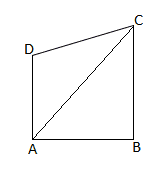Sol:

Given: ABCD is a quadrilateral and AC is one of its diagonals

(i) In ΔABC$\Delta ABC$, AB + BC > AC . . . . . . . . (1)

In ΔACD$\Delta ACD$, CD + DA > AC . . . . . . . . . . (2)

Adding inequalities (1) and (2), we get:

AB + BC + CD + DA > 2AC

(ii) In ΔABC$\Delta ABC$, we have :

AB + BC > AC . . . . . . . . (1)

We also know that the length of each side of a triangle is greater than the positive difference of the the other two sides.

In ΔACD$\Delta ACD$, we have:

AC > |DACD|$\left | DA – CD \right |$ . . . . . . . (2)

From (1) and (2), we have:

AB + BC > |DACD|$\left | DA – CD \right |$

$\Rightarrow$ AB + BC + CD > DA

(iii) In ΔABC$\Delta ABC$, AB + BC > AC

In ΔACD$\Delta ACD$, CD + DA > AC

ΔBCD$\Delta BCD$, BC + CD > BD

ΔABD$\Delta ABD$, DA + AB > BD

Adding these inequalities, we get:

2 (AB + BC + CD + DA) > 2 (AC + BD)

$\Rightarrow$ (AB + BC + CD + DA) > (AC + BD)

Q.10: Prove that the sum of all angles of a quadrilateral is 360°.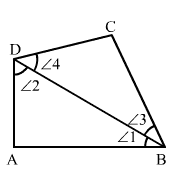Sol: Let ABCD be a quadrilateral and ∠1, ∠2, ∠3 and ∠4 are its four angles as shown in the figure.

Join BD which divides ABCD in two triangles, ΔABD$\Delta ABD$ and ΔBCD$\Delta BCD$.

In ΔABD$\Delta ABD$, we have:

∠ 1 + ∠ 2 +  ∠ A = 180° . . . . . . . . (i)

In ΔBCD$\Delta BCD$, we have:

∠ 3 + ∠ 4 + ∠ C = 180° . . . . . . . (ii)

On adding (i) and (ii), we get:

(∠1 + ∠3) + ∠A + ∠C + (∠ 4 + ∠2) = 360°

$\Rightarrow$ ∠ A + ∠ C + ∠ B + ∠ D = 360°   ( Since,∠ 1 + ∠ 3 = ∠ B; ∠ 4 + ∠ 2 = ∠ D)

∴ ∠ A + ∠ C + ∠ B + ∠ D = 360°

### RS Aggarwal Class 9 Solutions Chapter 9 – Quadrilaterals And Parallelograms

The RS Aggarwal Class 9 Solutions provides all the answers in a proper stepwise method which makes you well versed with all the solutions including the tougher ones. If you want to score good marks in your Class 9 examination, then, practicing RS Aggarwal Class 9 Maths solutions is an utmost necessity. It is one of the best study material when it comes a providing a question bank to practice from and also test the students understanding of concepts.